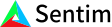1. Bob Anderson
×
IntuitiveML

## Intuition: How does the Heaviside Activation Function work?

Jun 11, 2020

Early on in the development of neural networks, most activation functions were created to represent the action potential firing in a neuron, because after all, neural networks were originally inspired by how the brain works.

The easiest way to represent the action potential is by having a function that is either active or not, that is, zero if the neuron isn’t active and one if it is active. That is called the Heaviside step function. The problem we have here is that gradient based methods can’t use it to learn because it’s not differentiable at 0 and the slope is 0 at all other values. We can try to fix this by modifying it so that instead of having flat lines, the lines have a small slope, like this. However, it turns out to that because this has the same slope the whole way through except for right here at the jump at x=0, this is functionally equivalent to using a linear activation function – that is, if you use this activation function for your whole neural network the output will be a linear combination of the inputs.

This is less than ideal, as not all functions are linear, so we want a function that looks kind of like the Heaviside step function, is nonlinear, and is differentiable at all points. AKA, we want something that looks like this. There are a few different functions that look like this and have these properties, but the most commonly used one would be the sigmoid function.